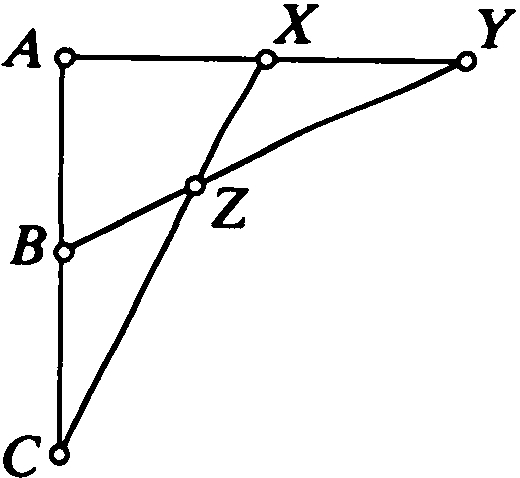## LogicThis diagram portrays the famous Group Configuration. In a stable structure, every such combinatorial configuration comes from a definable group.© 2021

Set theory and model theory, which are the principle areas of mathematical logic represented in Münster, concern themselves in different ways with fundamental questions of mathematics. The first studies natural models of set theory and the interplay between the existence of large cardinals, properties of definable sets of real numbers, combinatorial principles, and the cardinalities of specific sets. In model theory, one studies mathematical objects (groups, fields, geometries, ...) and examines their theories and the structure of their definable sets, considering properties such as stability. We analyse models of a theory, and use structural properties to better understand these models and to construct new examples.

# Prerequisites

The courses on Mathematical Logic I and Mathematical Logic II with the following contents:

Methods of model construction, the Gödel completeness theorem, undecidability and the Gödel incompleteness theorems, axiomatic set theory, ordinals and cardinals.

Model theory has strong connections to algebra. Knowledge of algebra is therefore desirable for students who plan to specialise in model theory.

# Courses for the Specialisation in "Logic"

Winter semester 2020/2021

Prof. Dr. Ralf Schindler: Mathematical Logic III - Advanced Set Theory (Type I)

Summer semester 2021

Prof. Dr. Ralf Schindler: Logic 4, Introduction to set theory, part 2 (Type II)
Prof. Dr. Dr. Katrin Tent: Introduction to Model Theory (Type I, II)

Winter semester 2021/2022

Prof. Dr. Aleksandra Kwiatkowska: "Logic 3: Descriptive Set Theory" (Type I)
Prof. Dr. Ralf Schindler: "Selected Topics from Set Theory" (Type II)
Prof. Dr. Dr. Katrin Tent: "Geometric Group Theory II" (Type I, II)

Abstract for the course of Prof. Dr. Ralf Schindler "Selected Topics from Set Theory": We will introduce and apply various important forcing notions, namely the stationary tower forcing, the extender algebra, as well as forcings which get used in the context of forcing axioms including the souped up version of Jensen's L-forcing which proves MM++ => (*). We presuppose only basic knowledge of forcing, all the relevant more specialized concepts will be presented in the course.

Type I lecture courses may be taken as "Mathematical Logic 3", Type II as "Mathematical Logic 4".

# Further information

The subject areas of the research groups are the following:

Further information regarding lecture courses and student seminars which are offered in mathematical logic, as well as research seminars, master theses etc., can be found on the websites of these research groups.